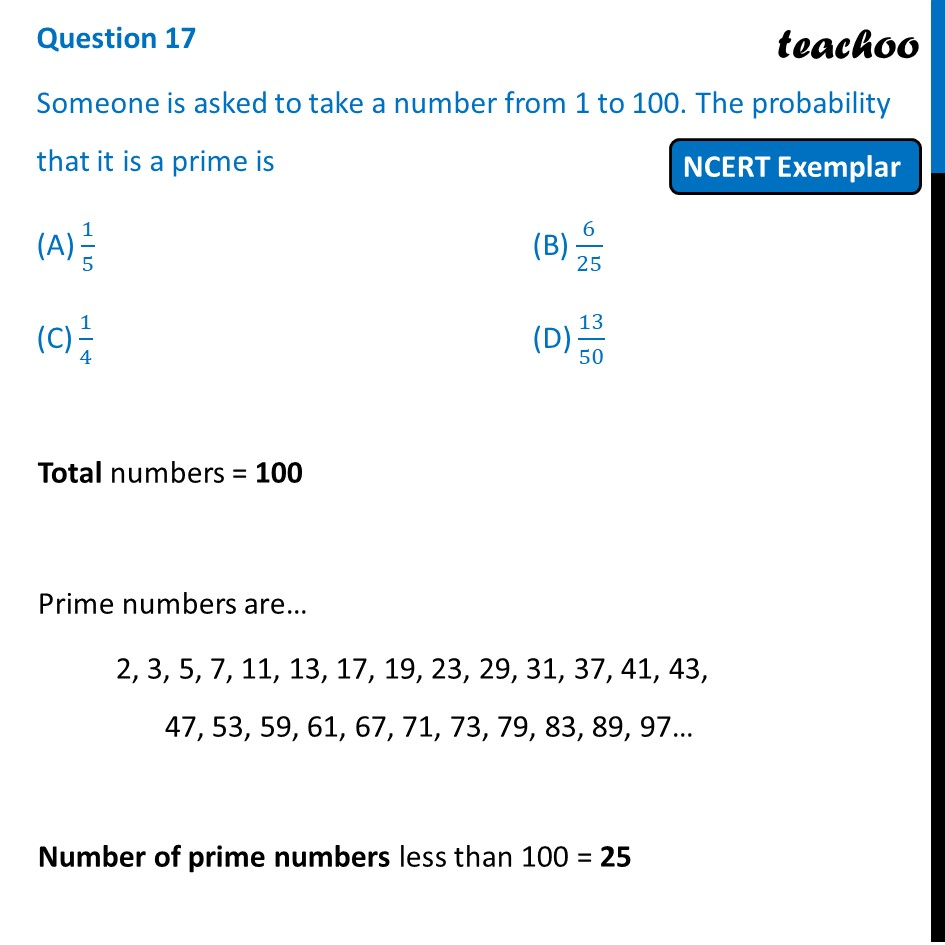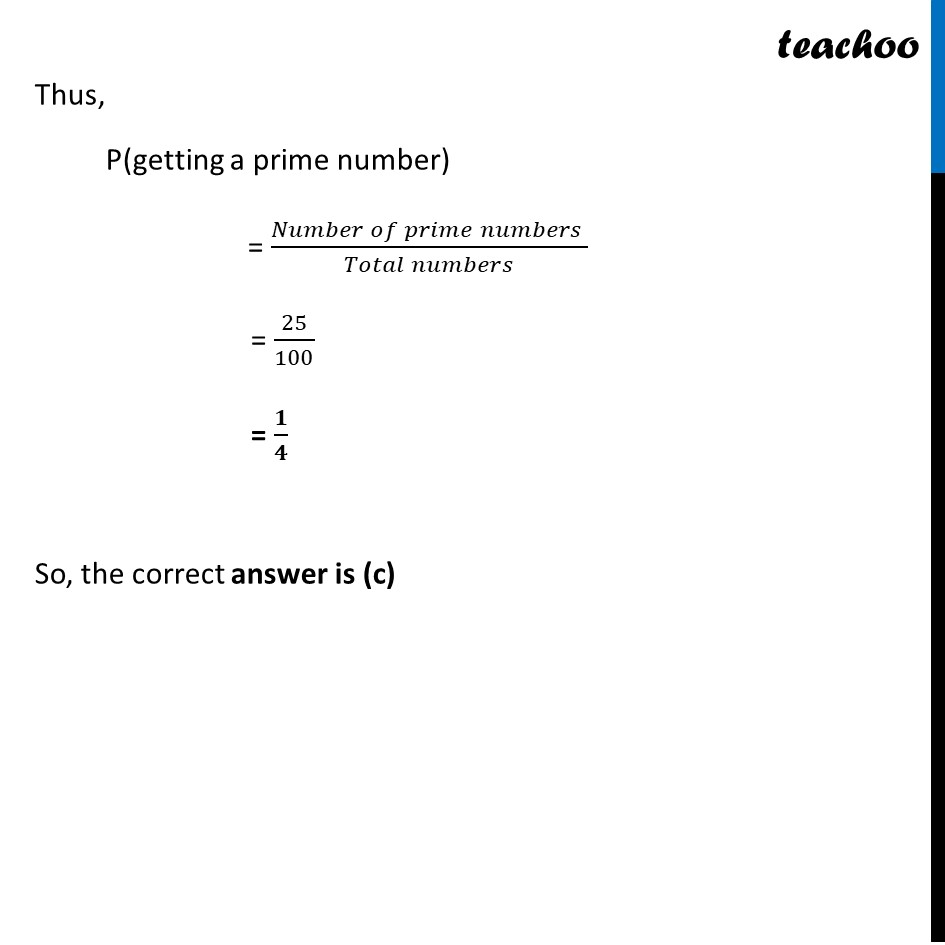NCERT Exemplar - MCQ

Chapter 14 Class 10 Probability
Serial order wise

## (C) 1/4   (D) 13/50Learn in your speed, with individual attention - Teachoo Maths 1-on-1 Class

### Transcript

Question 17 Someone is asked to take a number from 1 to 100. The probability that it is a prime is (A) 1/5 (B) 6/25 (C) 1/4 (D) 13/50 Total numbers = 100 Prime numbers are… 2, 3, 5, 7, 11, 13, 17, 19, 23, 29, 31, 37, 41, 43, 47, 53, 59, 61, 67, 71, 73, 79, 83, 89, 97… Number of prime numbers less than 100 = 25 Thus, P(getting a prime number) = (𝑁𝑢𝑚𝑏𝑒𝑟 𝑜𝑓 𝑝𝑟𝑖𝑚𝑒 𝑛𝑢𝑚𝑏𝑒𝑟𝑠 )/(𝑇𝑜𝑡𝑎𝑙 𝑛𝑢𝑚𝑏𝑒𝑟𝑠) = 25/100 = 𝟏/𝟒 So, the correct answer is (c)